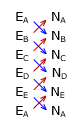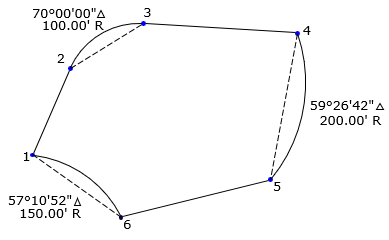### 1. Traverse with straight sides

#### a. Closed polygon

The area of any closed non-crossing polygon, Figure H-1,Figure H-1 Closed non-crossing polygons

can be computed using the coordinates of its verticies with Equation H-1:Equation H-1

This equation works for any polygon with straight sides. The more verticies, the more terms in the equation. An easy way to remember equations is graphically:

 Starting at one point, list the coordinates in sequence around the exterior. Repeat the first point at the end.Cross-multiply the coordinates.Sum the cross-products.Subtract one sum from the other, divide the result by two, and take the absolute value. This is the polygon area.Equation H-2

It doesn't matter:

• at which point you start
• going clockwise or counterclockwise around the polygon
• whether coordinates are East-North or North-East

The last two can affect the area's mathematical sign which is why Equation H-2 uses the absolute value.

#### b. Example area computation

Determine the area of the traverse in Figure H-2.Figure H-2 Area Example

Set up the coordinates table with additional rows for first point repetition and sums and two columns for cross-products.

We'll start at point C, travel clockwise, and carry an extra significant figure to minimize cumulative rounding.

 Point North (ft) East (ft)C 406.31 1259.97 D 235.12 1489.47 E 65.81 1126.40 A 317.89 942.04 B 675.32 1282.54 C 406.31 1259.97 sums:

Partial cross-products:406.31 x 1489.47 = 605,187
235.12 x 1126.40 = 264,489
...235.12 x 1259.97 = 296,244
65.81 x 1489.47 = 98,022
...

The units on the cross-products are square feet.

Completed table:

 Point North (ft) East (ft)C 406.31 1259.97 296,244 D 235.12 1489.47 605,187 98,022 E 65.81 1126.40 264,839 358,071 A 317.89 942.04 61,996 636,178 B 675.32 1282.54 407,707 521,109 C 406.31 1259.97 850,883 sums: 2,190,612 1,909,624Since we carried an extra significant figure, Area = 140,490 sq ft.

#### c. Non-crossing traverses only

Equation H-1 will not return a correct area if a traverse crosses itself. The traverse in Figure H-3 represents the order in which the points were surveyed, traverse adjusted, etc. Applying Equation H-1 to the coordinates in their surveyed order results in an "area" of 8,412 sq ft. The area is nonsensical since the traverse doesn't have an "inside" like a non-crossing polygon.Coordinates

 Point N (ft) E (ft) A 1000.00 2000.00 B 1248.80 1881.25 C 1019.65 1607.03 D 1217.88 1643.52

Area ABCDA = 8,412 sq ft.

Figure H-3
Crossing traverse

If we re-order the point list to a non-crossing perimeter, Figure H-4, the area is 70,717 sq ft.Coordinates

 Point N (ft) E (ft) A 1000.00 2000.00 C 1019.65 1607.03 D 1217.88 1643.52 B 1248.80 1881.25

Area ACDBA = 70,717 sq ft.

Figure H-4
Crossing traverse

Apply Equation H-1 only to a non-crossing traverse with the coordinates listed in order around the perimeter of the desired area.

### 2. Traverse with curves

#### a. Segments and sectors

Area by coordinates is constrained to following straight lines. Where curves are involved, Figure H-3(a), the method returns an area bounded by their chords, Figure H-3(b).(a) Traverse(b) Area by coordinates Figure H-3 Traverse with curves

To obtain the correct area, sector or segment areas are computed and added or subtracted accordingly. For the traverse in Figure H-3(a), the two segment areas are computed, Figure H-4(a),  and one subtracted from, the other added to, the area by coordinates, Figure H-4(b).(a) Segments(b) ATotal=A1-A2+A3 Figure H-4 Accounting for segments

#### b. Example area computation

Given the traverse in Figure H-5, determine its area.Figure H-5 Traverse with curves

In the table below, the points and their coordinates are in the first three columns; their cross-products are in the last two.

 Point North (ft) East (ft)1 500.00 1200.00 737,634 2 614.70 1249.70 624,850. 843,243 3 674.76 1347.43 828,261 892,620. 4 662.46 1552.76 1,047,730 725,778 5 467.41 1516.87 1,004,860 633,075 6 417.36 1317.39 615,766 658,696 1 500.00 1200.00 500,828 sums: 4,491,046 4,622,300.

The area isThis is the area bounded by the chords. We must account for the segments.

Recall that the area of a segment, bounded by an arc and its chord, isEquation H-2

Compute the area of each segment

Arc 2-3Arc 4-5Arc 6-1Total traverse area is 68,790 sq ft.

#### c. Segment or sector?

Consider the curvinlinear traverse in Figure H-5:Figure H-5 Curvilinear traverse

Its area can be computed using the arc sector, Figure H-6,(a) Area to radial lines, AR (b) Sector area, ASec Figure H-6 Area = AR + ASec

or using the arc segment, Figure H-7(a) Area to chord, AC (b) Segment area, ASeg Figure H-7 Area = AC + ASeg

Either method is fine. The only difference is that sectors require computing arc radius points, Figure H-6(a); segments do not, Figure H-7(a).